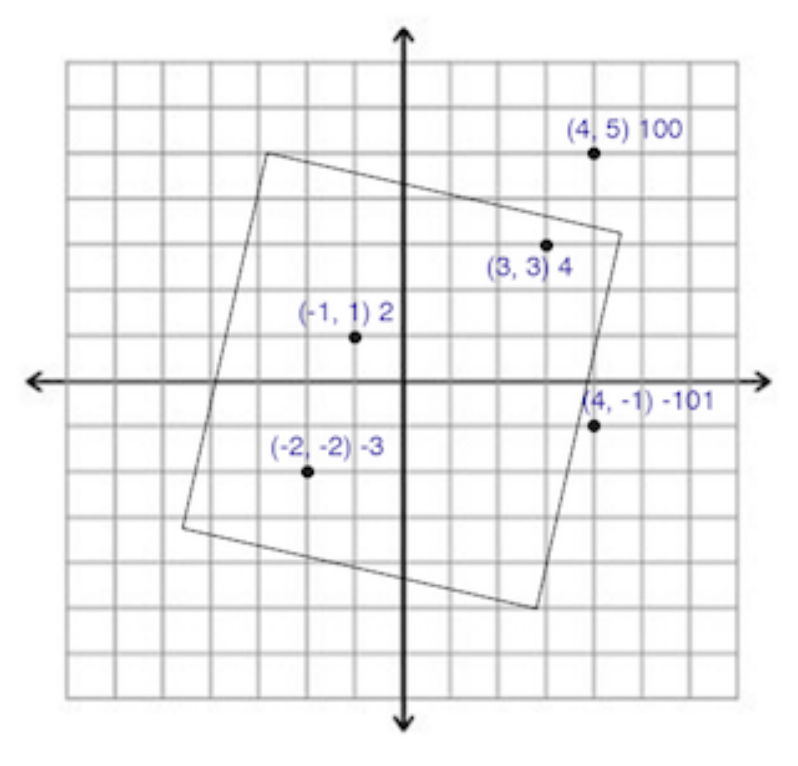시간 제한 메모리 제한 제출 정답 맞은 사람 정답 비율
2 초 512 MB 0 0 0 0.000%

## 문제

Darts is a fun game, in the game you shoot several darts on a board and you get a score for each dart based on its location. The game in this problem is a bit different.

The board in this problem can be considered as an infinite 2-dimensional plane, and you have already shot N darts. For each dart, you are given its location on the board as a point (X, Y ), and also for each dart you are given a score which you will get if we count this dart, this score can be negative or positive, and multiple darts can be at the exact same location with even different scores.

Here’s how the scoring happens. After shooting the N darts, you are required to draw a square on this plane, the length of the square side must be L, also the point (0, 0) must be exactly at the center of the square, but you can rotate the square in any way you want. Then you will get the score for each dart which is inside that square or on its boundaries.

Note that you can rotate the square only once before considering any darts.

Given the location and the score for each dart and the value of L, your task is to draw a square that gives you the maximum possible score (it’s okay if the square doesn’t contain any darts).

## 입력

Your program will be tested on one or more test cases. The first line of the input will be a single integer T (1 ≤ T ≤ 100) representing the number of test cases. Followed by T test cases.

Each test case starts with a line containing an integer N (1 ≤ N ≤ 105) representing the number of darts, followed by a space then an integer L (1 ≤ L ≤ 105) which is the length of the square side.

Followed by N lines, each line will contain 3 integers separated by a space, ‘X Y S’, which means there’s a dart at point (X, Y) and will give you score S if it’s counted (−105 ≤ X, Y, S ≤ 105).

## 출력

For each test case print a single line containing the maximum score you can get after drawing the square.

## 예제 입력 1

2
5 8
4 5 100
3 3 4
-2 -2 -3
-1 1 2
4 -1 -101
7 10
1 1 -10
-1 1 -10
1 -1 -10
-1 -1 -10
0 1 -10
1 0 -10
2 2 -1000


## 예제 출력 1

3
-1060


## 힌트

The following image represents the first sample test case, there’s no way to take the point which gives score 100, but we can rotate the square little bit such that it no longer includes the point which gives score -101.